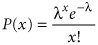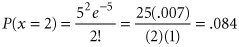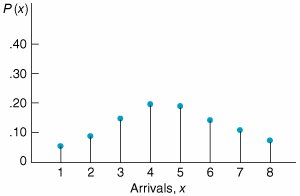# The Poisson Distribution

[Page 779 ( continued )]

The formula for a Poisson distribution iswhere

l = average arrival rate (i.e., arrivals during a specified period of time)

x = number of arrivals during the specified time period

e = 2.71828

x ! = the factorial of a value, x

[i.e., x ! = x ( x 1) ( x 2) . . . (3) (2) (1)]

As an example of this distribution, consider a service facility that has an average arrival rate of five customers per hour ( l = 5). The probability that exactly two customers will arrive at the service facility is found by letting x = 2 in the preceding Poisson formula:The value .084 is the probability of exactly two customers' arriving at the service facility.

By substituting values of x into the Poisson formula, we can develop a distribution of customer arrivals during a 1-hour period, as shown in Figure C.1. However, remember that this distribution is for an arrival rate of five customers per hour. Other values of l will result in distributions different from the one in Figure C.1.

##### Figure C.1. Poisson distribution for l = 5Introduction to Management Science (10th Edition)
ISBN: 0136064361
EAN: 2147483647
Year: 2006
Pages: 358

Similar book on Amazon

flylib.com © 2008-2017.
If you may any questions please contact us: flylib@qtcs.net